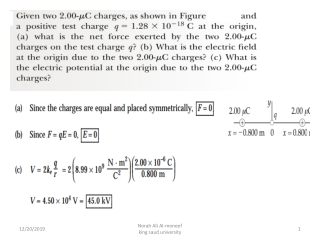Download Presentationq1

Loading in 2 Seconds...

# q1

Download Presentation## q1

- - - - - - - - - - - - - - - - - - - - - - - - - - - E N D - - - - - - - - - - - - - - - - - - - - - - - - - - -
##### Presentation Transcript

1. Norah Ali Al-moneef king saud university

2. Norah Ali Al-moneef king saud university

3. Norah Ali Al-moneef king saud university

4. q1 q2 d d P d d q4 q3 q1 = +12 n C, q2= -24 n C q3 = +31 n C, q4= +17 n C What is the potential at point P, located at the center of the square of point charges. Assume that d = 1.3m and the charges are Norah Ali Al-moneef king saud university

5. Two point charges each of magnitude 2.00 μC are located on the x axis. One is at x = 1.00 m, and the other is at x = ‑1.00 m. a) Determine the electric potential on the y axis at y = 0.500 m. b) Calculate the change in electric potential energy of the system as a third charge of –3.00 μC is brought from infinitely far away to a position on the y axis at y = 0.500 m. Norah Ali Al-moneef king saud university

6. A proton is moved from the negative plate to the positive plate of a parallel-plate arrangement. The plates are 1.5cm apart, and the electric field is uniform with a magnitude of 1500N/C. • How much work would be required to move a proton from the negative to the positive plate? • What is the potential difference between the plates? • If the proton is released from rest at the positive plate, what speed will it have just before it hits the negative plate? Norah Ali Al-moneef king saud university

7. A uniform electric field of magnitude 290 V/m is directed in the positive x direction. A +13.0 µC charge moves from the origin to the point (x, y) = (20.0 cm, 50.0 cm).(a) What is the change in the potential energy of the charge field system? Norah Ali Al-moneef king saud university

8. Gain or loss? A negative charge gains electric potential energy when it moves in the direction of the electric field. Explain this. A proton loses potential energy when moving in the direction of the electric field, but picks up an equal amount of kinetic energy. Work done by a field is positive when energy is given to an object from the field (object is lowered in a gravitational field or moved in the direction of E filed lines). This occurs when Uf <Uiso, W=-ΔU

9. 1- The electric field has a magnitude of 3.0 N/m at a distance of 60 cm from a point charge. What is the charge? (a) 1.4 nC (b) 120 pC (c) 36 mC (d) 12 C (e) 3.0 nC Norah Ali Al-moneef king saud university

10. The gap between electrodes in a spark plug is 0.06cm, To produce an electric spark in a gasoline air mixture, an E field of 3x106V/m must be achieved. When starting the car, what minimum potential difference must be supplied by the ignition circuit?

11. Calculate the speed of a proton that is accelerated from rest through a potential difference of 120V. An electron?

12. An ion accelerated through a potential difference of 115V experiences an increase in kinetic energy of 7.37x10-17 J.Calculate the charge of the ion

13. How much work is done in moving Avogadro's number of electrons from an initial point where the electric potential is 9V to a point where the potential is -5V?

14. Compute the energy necessary to bring together the charges in the configuration shown below: Calculate the electric potential energy between each pair of charges and add them together.

15. What is the electric potential at the point • i) 10 cm and • ii) 50 cm from a point charge of 2 µC ? • Find the work done to move a charge of 0.05 µC from • a point 50 cm from the point charge 2 µC to a point 10 cm • from the point charge. State whether the work done by • or on the electric field. a) b) Work done on the electric field.

16. Two point charges, 8x10-9 C and -2x10-9 C are separated by 4 m. The electric field magnitude (in units of V/m) midway between them is: • 9x109 B) 13,500 C) 135,000 D) 36x10-9 E) 22.5 Norah Ali Al-moneef king saud university